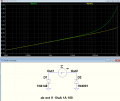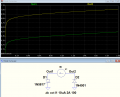# Measure Diode Resistence

#### kalemaxon89

Joined Oct 12, 2022
158
I measured in the lab the forward voltage of a diode by running different current values on it and plotted the I-V characteristic on excell.
I would like to calculate the resistance of the diode and would like to know if the reasoning I have done is correct:
- the resistance is the inverse of the slope of the I-V curve (inverse because the current is in the ordinates and the voltage is in the abscissas) .. i.e. on Excell I will calculate the resistance as =1/SLOPE(y_values ; x_values) .. so 1/SLOPE(current_values ; voltage_values) where current_values and voltage_values are all values measured in the lab.
The scalar number I get is therefore the resistance of the diode (in formward conduction)?
Am I doing something wrong?

#### LvW

Joined Jun 13, 2013
1,646
In case of a non-linear relationship between I and V you always have to discriminate between (a) the static and (b) the dynamic (differential) resistance.
In case (a) you have to analyze the slope of the line between the origin and the corresponding working point
In case (b) - as you wrote - you have to analyze the slope of the tangent at the working point.

•kalemaxon89

#### StefanZe

Joined Nov 6, 2019
158
Hi,
because the I-V curve is not linear you have to types of resistance. The static (or DC) resistance and the dynamic (or AC) resistance.
The static resitance is just V/I and the dynamic resistance is dV/dI.

•kalemaxon89

#### crutschow

Joined Mar 14, 2008
32,064
If you are looking for the parasitic ohmic resistance of the diode, that's the difference between its theoretical voltage drop and it's actual voltage drop at high currents.
For example, below is the simulation of the forward voltage of a 1N4148 diode and a 1N4001 diode versus forward current.
Since current is plotted on a log scale, the straight line shows the normal logarithmic relation between current and voltage for a diode.
The deviation from the straight line at higher currents is due to the intrinsic ohmic resistance of the diode.
Note that the deviation is much greater for the smaller 200mA, 1N4848 signal diode (green trace), versus the 1A, 1N4001 rectifier diode (yellow trace), as would be expected.
The dv/dI of the curve at the high current will give the value of that resistance (to a close approximation).

To avoid heating of the diode due to the current affecting your measurements, they should be done with a short pulse of current.#### kalemaxon89

Joined Oct 12, 2022
158
If you are looking for the parasitic ohmic resistance of the diode, that's the difference between its theoretical voltage drop and it's actual voltage drop at high currents.
For example, below is the simulation of the forward voltage of a 1N4148 diode and a 1N4001 diode versus forward current.
Since current is plotted on a log scale, the straight line shows the normal logarithmic relation between current and voltage for a diode.
The deviation from the straight line at higher currents is due to the intrinsic ohmic resistance of the diode.
Note that the deviation is much greater for the smaller 200mA, 1N4848 signal diode (green trace), versus the 1A, 1N4001 rectifier diode (yellow trace), as would be expected.
The dv/dI of the curve at the high current will give the value of that resistance (to a close approximation).

To avoid heating of the diode due to the current affecting your measurements, they should be done with a short pulse of current.

View attachment 290324
I would also like to simulate these two curves but I am using the two diodes 1N40xx and 1N58xx. They are not on my LTSpice. Although unrelated to the question I asked, if you have the LTSpice models of these 2 diodes, can you attach them to me? I went crazy looking for them and couldn't find it.

Therefore, in the curves you showed me, if I want to calculate the diode resistance I should do as @LvW suggested:
- Static R: analyse the slope of the line between the origin and the corresponding operating point
- R dynamic: analyse the slope of the tangent to the operating point
Am I wrong?

#### BobTPH

Joined Jun 5, 2013
6,523
Neither if those is actually a resistance. Yes, they are a ratio of current to voltage, but they differ from each other and at each point on the curve. A resistance is a constant over a wide range of voltages and currents.

•kalemaxon89

#### crutschow

Joined Mar 14, 2008
32,064
I would also like to simulate these two curves but I am using the two diodes 1N40xx and 1N58xx.
See below.
The xx represents any diode in the series (e.g. 1N4001 to 1N4007), which are the same expect being sorted for reverse voltage rating.
If you don't have them, I can post them.
A resistance is a constant over a wide range of voltages and currents.
The slope at the high current levels, where the resistance becomes dominant, will have a relatively constant resistance value from the dv/di calculation.
Below is a linear plot that shows pretty close to a straight line at the high current levels, indicating that it is due to a predominately constant ohmic resistance.#### LvW

Joined Jun 13, 2013
1,646
Neither if those is actually a resistance. Yes, they are a ratio of current to voltage, but they differ from each other and at each point on the curve. A resistance is a constant over a wide range of voltages and currents.
What about the differential (dynamic) voltage-to-current ratio of a non-linear characteristic?
Dont we call it resistance? Remember the parameters of a Z.diode (r_z).
And what about h11=hie for a bipolar transistor ?
Dont we speak about the input resistance of the device?
Of course, the term "resistor" was introduced long time ago for a part which fulfills the conditions you have mentioned ("constant over a wide range...").
However, in the following years, many new components with non-linear properties were developed. Why should one not be able to make use of the introduced term "resistance" for the voltage-current ratios, even when they are not constant?

In this context, there is one case I totally agree with you.
Some people use the term "internal emitter resistance" for the inverse transconductance gm of a bipolar transistor (re=1/gm).
This is totally wrong! This is not a resistance (not a two-pole parameter) and it is not "internal" to the emitter.
Even worse - such a wrong "definition" leads to a false understanding how the BJT really works.

Last edited:

#### kalemaxon89

Joined Oct 12, 2022
158
See below.
The xx represents any diode in the series (e.g. 1N4001 to 1N4007), which are the same expect being sorted for reverse voltage rating.
If you don't have them, I can post them.
The slope at the high current levels, where the resistance becomes dominant, will have a relatively constant resistance value from the dv/di calculation.
Below is a linear plot that shows pretty close to a straight line at the high current levels, indicating that it is due to a predominately constant ohmic resistance.

View attachment 290338
The diode I need are 1N5819 and 1N4004 because I used those in the lab measurements.
If you don't have those specifically, other similar ones from the same family are still OK.
If you can publish them thank you very much!

Last edited:

#### BobTPH

Joined Jun 5, 2013
6,523
What about the differential (dynamic) voltage-to-current ratio of a non-linear characteristic?
Dont we call it resistance? Remember the parameters of a Z.diode (r_z).
And what about h11=hie for a bipolar transistor ?
Dont we speak about the input resistance of the device?
Of course, the term "resistor" was introduced long time ago for a part which fulfills the conditions you have mentioned ("constant over a wide range...").
However, in the following years, many new components with non-linear properties were developed. Why should one not be able to make use of the introduced term "resistance" for the voltage-current ratios, even when they are not constant?

In this context, there is one case I totally agree with you.
Some people use the term "internal emitter resistance" for the inverse transconductance gm of a bipolar transistor (re=1/gm).
This is totally wrong! This is not a resistance (not a two-pole parameter) and it is not "internal" to the emitter.
Even worse - such a wrong "definition" leads to a false understanding how the BJT really works.
Because if you just use the term "resistance", as the TS did, then it might mean one of three things. It has to be qualified to have an unambiguous meaning.

#### BobTPH

Joined Jun 5, 2013
6,523
To the TS: What do you intend to do with the resistance measurement you come up with? In two of the cases, it applies only to a specific point on the IV curve, and therefore cannot be used to calculate anything other than the small signal behavior in the case of "dynamic resistance." And the third, as described by @MrChips, is meaningful only in an area where the diode should never be operated.

#### crutschow

Joined Mar 14, 2008
32,064
Below is a zip file containing the standard.dio file with the diodes you wanted, which goes in the LTspice CMP folder.

#### Attachments

• 3.6 KB Views: 1
•kalemaxon89

#### kalemaxon89

Joined Oct 12, 2022
158
See below.
The xx represents any diode in the series (e.g. 1N4001 to 1N4007), which are the same expect being sorted for reverse voltage rating.
If you don't have them, I can post them.
The slope at the high current levels, where the resistance becomes dominant, will have a relatively constant resistance value from the dv/di calculation.
Below is a linear plot that shows pretty close to a straight line at the high current levels, indicating that it is due to a predominately constant ohmic resistance.

View attachment 290338
Thanks! I will try to simulate

Last edited:

#### LvW

Joined Jun 13, 2013
1,646
Because if you just use the term "resistance", as the TS did, then it might mean one of three things. It has to be qualified to have an unambiguous meaning.
Yes - and this was the background for my first answer (see post#9).